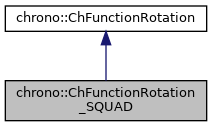## Description

A rotation function q=f(s) that interpolates n rotations using a SQUAD spherical quadrangle interpolation between quaternions.

Differently from the ChFunctionRotation_spline of order 3, this cubic interpolation really passes through the control points. In the original single-span SQUAD algorithm, 4 quaternions are used: the interpolation passes exactly in 1st and 4th, whereas 2nd and 3rd are 'magnetic' as ctrl points in splines; in our implementation the 2nd and 3rd are computed automatically given the sequence of the many SQUAD spans, per each span, enforcing continuity inter span.

#include <ChFunctionRotation_SQUAD.h>[legend][legend]

## Public Member Functions

Constructor. By default constructs a linear SLERP between two identical null rotations.

ChFunctionRotation_SQUAD (const std::vector< ChQuaternion<> > &mrotations, ChVectorDynamic<> *mknots=0)
Constructor from a given array of control points; each control point is a rotation to interpolate. More...

"Virtual" copy constructor.

double ComputeUfromKnotU (const double u) const
When using Evaluate() etc. More...

double ComputeKnotUfromU (const double U) const
When using Evaluate() etc. More...

std::vector< ChQuaternion<> > & Rotations ()
Access the rotations, ie. quaternion SQUAD control points.

ChVectorDynamicKnots ()
Access the knots.

int GetOrder ()
Get the order of spline.

virtual void SetupData (const std::vector< ChQuaternion<> > &mrotations, ChVectorDynamic<> *mknots=0)
Initial easy setup from a given array of rotations (quaternion control points). More...

std::shared_ptr< ChFunctionGetSpaceFunction () const
Gets the address of the function u=u(s) telling how the curvilinear parameter u of the spline changes in s (time).

void SetSpaceFunction (std::shared_ptr< ChFunction > m_funct)
Sets the function u=u(s) telling how the curvilinear parameter of the spline changes in s (time). More...

void SetClosed (bool mc)
Set as closed periodic spline: start and end rotations will match at 0 and 1 abscyssa as q(0)=q(1), and the Evaluate() and Derive() functions will operate in periodic way (abscyssa greater than 1 or smaller than 0 will wrap to 0..1 range). More...

bool GetClosed ()
Tell if the rotation spline is closed periodic.

virtual ChQuaternion Get_q (double s) const override
Return the q value of the function, at s, as q=f(s). More...

virtual void ArchiveOUT (ChArchiveOut &marchive) override
Return the derivative of the rotation function, at s, expressed as angular velocity w in local frame. More...

virtual void ArchiveIN (ChArchiveIn &marchive) override
Method to allow de-serialization of transient data from archives.Public Member Functions inherited from chrono::ChFunctionRotation
ChFunctionRotation (const ChFunctionRotation &other)

virtual ChVector Get_w_loc (double s) const
Return the derivative of the rotation function, at s, expressed as angular velocity w in local frame. More...

virtual ChVector Get_a_loc (double s) const
Return the derivative of the rotation function, at s, expressed as angular acceleration in local frame. More...

virtual void Estimate_s_domain (double &smin, double &smax) const
Return an estimate of the domain of the function argument. More...

virtual void Update (const double t)
Update could be implemented by children classes, ex. to launch callbacks.

## Constructor & Destructor Documentation

 chrono::ChFunctionRotation_SQUAD::ChFunctionRotation_SQUAD ( const std::vector< ChQuaternion<> > & mrotations, ChVectorDynamic<> * mknots = 0 )

Constructor from a given array of control points; each control point is a rotation to interpolate.

Input data is copied. If the knots are not provided, a uniformly spaced knot vector is made.

Parameters
 mrotations rotations, to interpolate. Required: at least n = 2. mknots knots, as many as control points. If not provided, initialized to uniform.

## ◆ ArchiveOUT()

 void chrono::ChFunctionRotation_SQUAD::ArchiveOUT ( ChArchiveOut & marchive )
overridevirtual

Return the derivative of the rotation function, at s, expressed as angular velocity w in local frame.

Return the derivative of the rotation function, at s, expressed as angular acceleration in local frame. Method to allow serialization of transient data to archives

Reimplemented from chrono::ChFunctionRotation.

## ◆ ComputeKnotUfromU()

 double chrono::ChFunctionRotation_SQUAD::ComputeKnotUfromU ( const double U ) const
inline

When using Evaluate() etc.

you need U parameter to be in 0..1 range, but knot range is not necessarily in 0..1. So you can convert U->u, where u is in knot range, calling this:

## ◆ ComputeUfromKnotU()

 double chrono::ChFunctionRotation_SQUAD::ComputeUfromKnotU ( const double u ) const
inline

When using Evaluate() etc.

you need U parameter to be in 0..1 range, but knot range is not necessarily in 0..1. So you can convert u->U, where u is in knot range, calling this:

## ◆ Get_q()

 ChQuaternion chrono::ChFunctionRotation_SQUAD::Get_q ( double s ) const
overridevirtual

Return the q value of the function, at s, as q=f(s).

Parameter s always work in 0..1 range, even if knots are not in 0..1 range. So if you want to use s in knot range, use ComputeUfromKnotU().

Implements chrono::ChFunctionRotation.

## ◆ SetClosed()

 void chrono::ChFunctionRotation_SQUAD::SetClosed ( bool mc )

Set as closed periodic spline: start and end rotations will match at 0 and 1 abscyssa as q(0)=q(1), and the Evaluate() and Derive() functions will operate in periodic way (abscyssa greater than 1 or smaller than 0 will wrap to 0..1 range).

The closure will change the knot vector (multiple start end knots will be lost) and will create auxiliary p control points at the end that will be wrapped to the beginning control points.

## ◆ SetSpaceFunction()

 void chrono::ChFunctionRotation_SQUAD::SetSpaceFunction ( std::shared_ptr< ChFunction > m_funct )
inline

Sets the function u=u(s) telling how the curvilinear parameter of the spline changes in s (time).

Otherwise, by default, is a linear ramp, so evaluates the spline from begin at s=0 to end at s=1

## ◆ SetupData()

 void chrono::ChFunctionRotation_SQUAD::SetupData ( const std::vector< ChQuaternion<> > & mrotations, ChVectorDynamic<> * mknots = 0 )
virtual

Initial easy setup from a given array of rotations (quaternion control points).

Input data is copied. If the knots are not provided, a uniformly spaced knot vector is made.

Parameters
 mrotations rotations, to interpolate. Required: at least n = 2. mknots knots, as many as control points. If not provided, initialized to uniform.

The documentation for this class was generated from the following files: